• 代码： from sklearn import datasets from sklearn.linear_model import Linear...# 使用以后的数据集进行线性回归（这里是波士顿房价数据） ##1 数据的加载 from sklearn.model_selection import train_t...
代码：
from sklearn import datasets
from sklearn.linear_model import LinearRegression
import matplotlib.pyplot as plt

# 使用以后的数据集进行线性回归（这里是波士顿房价数据）
##1 数据的加载
from sklearn.model_selection import train_test_split

X_train,X_test,y_train,y_test=train_test_split(data_X,data_y,test_size=0.2,random_state=33)
##2 模型的加载
model = LinearRegression()

##3 模型的训练
model.fit(X_train, y_train)

print(model.score(X_test,y_test))


0.6923688459535136
调参：
LinearRegression(fit_intercept=True, normalize=False, copy_X=True, n_jobs=None) fit_intercept:是否有截据，如果没有则直线过原点; normalize:是否将数据归一化; copy_X:默认为True，当为True时，X会被copied,否则X将会被覆写; n_jobs:默认值为1。计算时使用的核数  
展开全文• 最近在写小论文，就是硕士毕业论文的部分成果，需要用到线性回归，所以就把相关代码贴上，便于日后写论文时总结，也顺便给后来者提供思路。小论文的思路主要是，先用线性回归，再用随机森林，最后再用xgboost，来...
一、前言
最近在写小论文，就是硕士毕业论文的部分成果，需要用到线性回归，所以就把相关代码贴上，便于日后写论文时总结，也顺便给后来者提供思路。小论文的思路主要是，先用线性回归，再用随机森林，最后再用xgboost，来预测房价，最后得出结论。所以，这个会陆续的贴出相关代码。至于数据清洗那一块，暂时不贴上了，主要原因就是数据清洗不容易，并且每个人的清洗方法也不一样，所以主要还是看思路。
二、算法简介
2.1 什么是回归分析
回归分析是一种预测性的建模技术，它研究的是因变量（目标）和自变量（预测器）之间的关系。这种技术通常用于预测分析，时间序列模型以及发现变量之间的因果关系。通常使用曲线/线来拟合数据点，目标是使曲线到数据点的距离差异最小。股票预测，房价预测等都属于回归分析，简单理解即：预测结果可以是任意的。和分类分析对比，分类就是对与错，1和0，是与否的关系，而回归问题则是结果不固定，可能是一个区间内的任意数字，比如房价，比如工资，可能是1-1w内的任意数字。
2.2 线性回归
线性回归是回归问题中的一种，线性回归假设目标值与特征之间线性相关，即满足一个多元一次方程。通过构建损失函数，来求解损失函数最小时的参数w和b。通长我们可以表达成如下公式：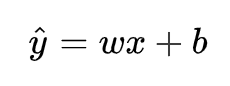y^为预测值，自变量x和因变量y是已知的，而我们想实现的是预测新增一个x，其对应的y是多少。因此，为了构建这个函数关系，目标是通过已知数据点，求解线性模型中w和b两个参数。
如果预测的是房价，最简单的理解，面积*单价=总价，但是单价是怎么来的呢？为啥有的地方可以卖到1w，有些地方能卖到3w呢，所以说房价的影响是多因素的。那么用线性回归来预测房价，只能说太过理想化或者简单化了。预测的结果肯定不会太高。
2.3 目标/损失函数
求解最佳参数，需要一个标准来对结果进行衡量，为此我们需要定量化一个目标函数式，使得计算机可以在求解过程中不断地优化。
针对任何模型求解问题，都是最终都是可以得到一组预测值y^ ，对比已有的真实值 y ，数据行数为 n ，可以将损失函数定义如下：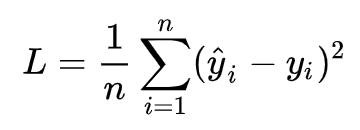即预测值与真实值之间的平均的平方距离，统计中一般称其为MAE(mean square error)均方误差。把之前的函数式代入损失函数，并且将需要求解的参数w和b看做是函数L的自变量，可得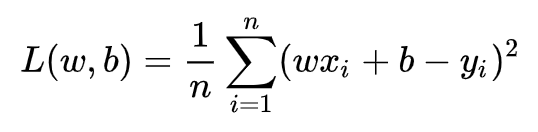现在的任务是求解最小化L时w和b的值，
即核心目标优化式为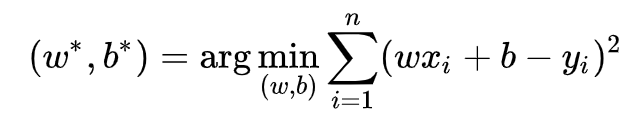求解方式有两种：
1）最小二乘法(least square method)
求解 w 和 b 是使损失函数最小化的过程，在统计中，称为线性回归模型的最小二乘“参数估计”(parameter estimation)。我们可以将 L(w,b) 分别对 w 和 b 求导，得到令上述两式为0，可得到 w 和 b 最优解的闭式(closed-form)解：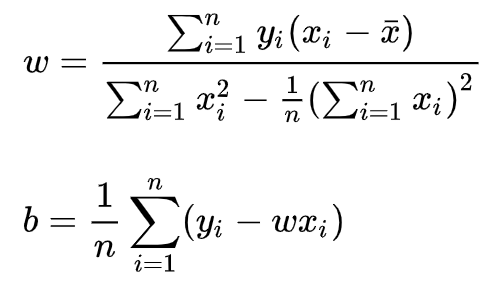梯度下降核心内容是对自变量进行不断的更新（针对w和b求偏导），使得目标函数不断逼近最小值的过程这里不做展开讲解。
三、代码及结果分析
from sklearn.impute import SimpleImputer
from sklearn.metrics import mean_absolute_error
from sklearn.model_selection import KFold
from sklearn.metrics import r2_score
from sklearn.preprocessing import *
from sklearn.model_selection import train_test_split
from sklearn.metrics import mean_squared_error
import importlib
import numpy as np
import matplotlib as mpl
import matplotlib.pyplot as plt
import pandas as pd
from sklearn import metrics
# 因为plt画图需要字体，所以下列三行是设置字体
from pylab import *
mpl.rcParams['font.sans-serif'] = ['SimHei']
mpl.rcParams['axes.unicode_minus'] = False

#用常数填充
df_merge = df_merge.replace(np.nan, 0)

# ****************线性回归***************************************************8
from sklearn.linear_model import LinearRegression
# 准备训练、测试集
X = df_merge.drop(['成交价'],axis=1)
y = df_merge['成交价']
x_train,x_test,y_train,y_test = train_test_split(X,y,test_size=0.2,random_state=42)    # random_state=42
x_train = x_train.astype(np.float64)
x_test = x_test.astype(np.float64)

# print(x_train.shape, x_test.shape, y_train.shape, y_test.shape)

# 设定回归算法
lr = LinearRegression()
# 使用训练数据进行参数求解
lr.fit(x_train, y_train)
# LinearRegression(copy_X=True, fit_intercept=True, n_jobs=1, normalize=False)
# print('求解系数为：', lr.intercept_)
# print('求解系数为：', lr.coef_)

# 对测试集的预测
y_hat = lr.predict(x_test)
# 打印前10个预测值
print("*"*10)
print("真实值：")
print(y_test[0:20])
print("预测值：")
print(y_hat[0:20])
# y_hat[0:9]
print("*"*10)

# y_test与y_hat的可视化
# 设置图片尺寸
plt.figure(figsize=(10, 6))
# 创建t变量
t = np.arange(len(x_test))
# 绘制y_test曲线
plt.plot(t, y_test, 'r', linewidth=2, label='真实值')
# 绘制y_hat曲线
plt.plot(t, y_hat, 'g', linewidth=2, label='预测值')
# 设置图例
plt.legend()
plt.show()

# 拟合优度R2的输出方法
print("r2:", lr.score(x_test, y_test))

# 用Scikit_learn计算MAE
print("MAE:", metrics.mean_absolute_error(y_test, y_hat))

# 用Scikit_learn计算MSE
print("MSE:", metrics.mean_squared_error(y_test, y_hat))

# 用Scikit_learn计算RMSE
print("RMSE:", np.sqrt(metrics.mean_squared_error(y_test, y_hat)))

结果
r2: 0.7374872620731299
MAE: 65.10673914738442
MSE: 8829.098955225081
RMSE: 93.96328514491753
运行时间： 0:00:00.299199 秒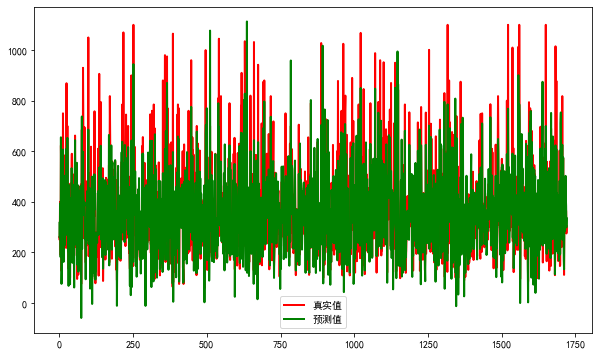调参：
LinearRegression(fit_intercept=True, normalize=False, copy_X=True, n_jobs=None) fit_intercept:是否有截据，如果没有则直线过原点; normalize:是否将数据归一化; copy_X:默认为True，当为True时，X会被copied,否则X将会被覆写; n_jobs:默认值为1。计算时使用的核数

分析：r2: 0.7374872620731299的值不算高，MSE: 8829.098955225081是挺高的，说明和预测值差距比较大。从图中也看出，好多的预测值偏低。毕竟线性回归本来就是毕竟简单的模型，也没有进行调参，那结果肯定可想而知。就像一个学生只知道学习，还是那种死学习，那他的成绩肯定高不到哪里去。下边的是预测值和真实值的比较。数据集大概是8k左右。有些还是比较准的，有些感觉就是瞎扯了。O(∩_∩)O哈哈~
    预测值      真实值
261.6408733    260
320.36511994    249
259.65389878    400
393.58315287    341
181.33705676    189
655.13792816    623
405.27933678    505
75.46051886    126
138.83768312    245
275.73318861    209
329.22648801    295
248.37913372    356
606.88028161    750
432.380307    400
162.20349804    177
453.66704175    344
299.03437647    216
285.30563981    284
228.46034506    245
271.25327428    345

展开全文机器学习
• 单变量线性回归 绘制散点图 相关系数R 拆分训练集和测试集 多变量线性回归 数据检验（判断是否可以做线性回归） 训练线性回归模型 先甩几个典型的线性回归的模型，帮助大家捡起那些年被忘记的数学。 ● 单...
目录
数据导入
单变量线性回归
绘制散点图
相关系数R
拆分训练集和测试集
多变量线性回归
数据检验（判断是否可以做线性回归）
训练线性回归模型

先甩几个典型的线性回归的模型，帮助大家捡起那些年被忘记的数学。
● 单变量线性回归： h(x)=theta0 + theta1* x 1 ● 多变量线性回归： h(x)=theta0 + theta1* x 1 + theta2* x 2 + theta3* x 3 ● 多项式回归：        h(x)=theta0 + theta1* x 1 + theta2* (x2^2) + theta3* (x3^3)
多项式回归始终还是线性回归，你可以令x2=x2^2，x3=x3^3，简单的数据处理一下就好了，这样上述多项式回归的模型就变成多变量线性回归的模型了。
数据导入
下面我们要开始用数据说话了，先来看看数据源是什么样子吧。
import pandas as pd
import numpy as np
import matplotlib.pyplot as plt
from pandas import DataFrame,Series
from sklearn.cross_validation import train_test_split
from sklearn.linear_model import LinearRegression

#读取文件
datafile = u'E:\\pythondata\\dhdhdh.xlsx'#文件所在位置，u为防止路径中有中文名称，此处没有，可以省略
examDf = DataFrame(data)
examDf.head()

运行结果：
     Call   Connect    Return
0  2.1335  1.267760  1.176615
1  2.5534  1.352941  1.978967
2  3.3361  1.872093  2.249511
3  3.3861  1.826667  2.986597
4  3.9682  1.875000  2.798570

单变量线性回归

绘制散点图
先将上述数据中的Connect（接通量）和Return（回款量）做一个单变量的线性回归，先绘制一个散点图，大致看一下分布情况。
#绘制散点图,examDf.jt为X轴，examDf.hk为Y轴
plt.scatter(examDf.Connect,examDf.Return,color = 'darkgreen',label = "Exam Data")

#添加图的标签（x轴，y轴）
plt.xlabel("The Connection amount of the average account")#设置X轴标签
plt.ylabel("The ratio of average return amount")#设置Y轴标签
plt.show()#显示图像

运行结果：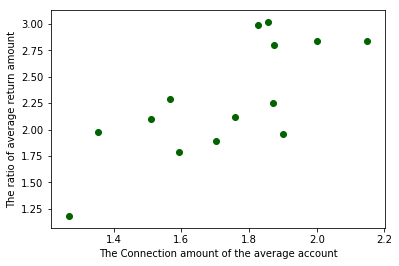看来商业中的实际数据总是和课本上的完美数据不一样，看这零零散散的分布，线性关系很是勉强，但是大致还是有一个线性相关的样子的，那么就看看相关系数有多少吧。
相关系数R
rDf = examDf.corr()#查看数据间的相关系数
print(rDf)

运行结果：
             Call   Connect    Return
Call     1.000000  0.445870  0.441673
Connect  0.445870  1.000000  0.745338
Return   0.441673  0.745338  1.000000

Connect（接通量）和Return（回款量）的相关系数为0.745338，还不错。
相关系数是用以反映变量之间相关关系密切程度的统计指标，对于相关性强度来说，我们一般认为：
0~0.3 弱相关
0.3~0.6  中等程度相关
0.6~1  强相关
在数学中，相关系数的计算一般是这样的，给数学狂人看：

R(相关系数) = X和Y的协方差 / (X的标准差 * Y的标准差) == cov（X,Y）/ σX * σY （即person系数）

拆分训练集和测试集
Connect（接通量）和Return（回款量）属于强相关，可以进行线性回归训练，那么我们先来拆分训练集和测试集吧。
#拆分训练集和测试集（train_test_split是存在与sklearn中的函数）
X_train,X_test,Y_train,Y_test = train_test_split(examDf.Connect,examDf.Return,train_size=0.8)
#train为训练数据,test为测试数据,examDf为源数据,train_size 规定了训练数据的占比

print("自变量---源数据:",examDf.Connect.shape, "；  训练集:",X_train.shape, "；  测试集:",X_test.shape)
print("因变量---源数据:",examDf.Return.shape, "；  训练集:",Y_train.shape, "；  测试集:",Y_test.shape)

#散点图
plt.scatter(X_train, Y_train, color="darkgreen", label="train data")#训练集为深绿色点
plt.scatter(X_test, Y_test, color="red", label="test data")#测试集为红色点

#添加标签
plt.legend(loc=2)#图标位于左上角，即第2象限，类似的，1为右上角，3为左下角，4为右下角
plt.xlabel("The Connection amount of the average account")#添加 X 轴名称
plt.ylabel("The ratio of average return amount")#添加 Y 轴名称
plt.show()#显示散点图

运行结果：
自变量---源数据: (14,) ；  训练集: (11,) ；  测试集: (3,)
因变量---源数据: (14,) ；  训练集: (11,) ；  测试集: (3,)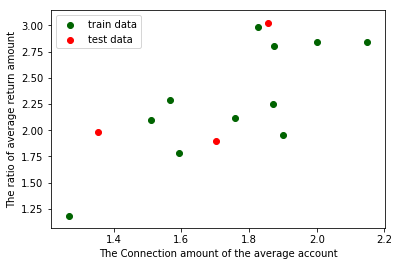回归模型训练
#调用线性规划包
model = LinearRegression()

#线性回归训练
model.fit(X_train,Y_train)#调用线性回归包

a  = model.intercept_#截距
b = model.coef_#回归系数

#训练数据的预测值
y_train_pred = model.predict(X_train)
#绘制最佳拟合线：标签用的是训练数据的预测值y_train_pred
plt.plot(X_train, y_train_pred, color='blue', linewidth=2, label="best line")

#测试数据散点图
plt.scatter(X_train, Y_train, color='darkgreen', label="train data")
plt.scatter(X_test, Y_test, color='red', label="test data")

#添加图标标签
plt.legend(loc=2)#图标位于左上角，即第2象限，类似的，1为右上角，3为左下角，4为右下角
plt.xlabel("The Connection amount of the average account")#添加 X 轴名称
plt.ylabel("The ratio of average return amount")#添加 Y 轴名称
plt.show()#显示图像

print("拟合参数:截距",a,",回归系数：",b)
print("最佳拟合线: Y = ",round(a,2),"+",round(b,2),"* X")#显示线性方程，并限制参数的小数位为两位


运行结果：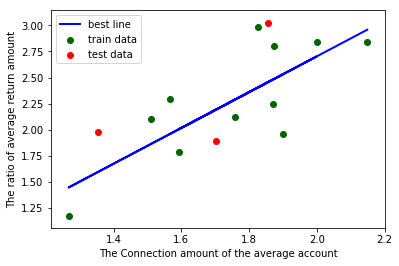拟合参数:截距 -0.7291766860887745 ,回归系数： [1.71584366]
最佳拟合线: Y =  -0.73 + 1.72 * X

补充一句，有博友说单变量线性回归上面的这段代码中，加一个reshape就能运行出来了，可能是版本不同，我没加也能运行出来，所以分享在下面给大家参考一下，如果有和博友一样的情况，可以试试增加下面一段代码（谢谢博友 dsy23 的分享）：

#调用线性规划包
model = LinearRegression()

#在这里加一段
X_train = X_train.values.reshape(-1,1)
X_test = X_test.values.reshape(-1,1)

#线性回归训练
model.fit(X_train,Y_train)#调用线性回归包

a  = model.intercept_#截距
b = model.coef_#回归系数

多变量线性回归
在单变量线性回归中，我们将每一步都讲解的极其详细，所以在多变量线性回归中，我们不会重复讲那些简单的部分了，但是为了防止python小白迷失自己，所以在这部分该有的代码还是会甩出来，该有的备注也都有，只不过不会一点一点分步骤来了。
上面我们提到多变量线性回归的模型为h(x)=theta0 + theta1* x 1 + theta2* x 2 + theta3* x 3，下面，我们还是使用单变量线性回归中的数据，单变量线性回归中，我们只用到了Connect（接通量）和Return（回款量），既然是多变量回归模型，那么我们就多加一个变量Call（拨打量）。

数据检验（判断是否可以做线性回归）
#-*- coding:utf-8 -*-

import pandas as pd
import numpy as np
import seaborn as sns
import matplotlib.pyplot as plt
from pandas import DataFrame,Series
from sklearn.cross_validation import train_test_split
from sklearn.linear_model import LinearRegression

#读取文件
datafile = u'E:\\pythondata\\dhdhdh.xlsx'#文件所在位置，u为防止路径中有中文名称，此处没有，可以省略
examDf = DataFrame(data)

#数据清洗,比如第一列有可能是日期，这样的话我们就只需要从第二列开始的数据，
#这个情况下，把下面中括号中的0改为1就好，要哪些列取哪些列
new_examDf = examDf.ix[:,0:]

#检验数据
print(new_examDf.describe())#数据描述，会显示最值，平均数等信息，可以简单判断数据中是否有异常值
print(new_examDf[new_examDf.isnull()==True].count())#检验缺失值，若输出为0，说明该列没有缺失值

#输出相关系数，判断是否值得做线性回归模型
print(new_examDf.corr())#0-0.3弱相关；0.3-0.6中相关；0.6-1强相关；

#通过seaborn添加一条最佳拟合直线和95%的置信带，直观判断相关关系
sns.pairplot(data, x_vars=['Call','Connect'], y_vars='Return', size=7, aspect=0.8, kind='reg')
plt.show()


运行结果：
            Call    Connect     Return
count  99.000000  99.000000  99.000000
mean    3.153649   1.967779   2.591121
std     1.027607   0.470364   0.790172
min     1.280100   1.014208   0.941292
25%     2.373528   1.596610   2.044147
50%     3.040000   1.913472   2.476091
75%     3.835980   2.253994   3.035603
max     5.851181   3.234977   5.160840
Call       0
Connect    0
Return     0
dtype: int64
Call   Connect    Return
Call     1.000000  0.837052  0.744792
Connect  0.837052  1.000000  0.844640
Return   0.744792  0.844640  1.000000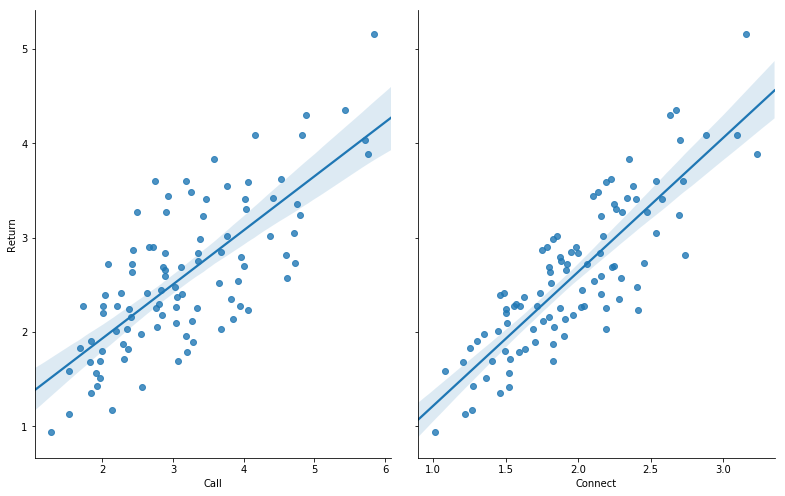判断是否可以做线性回归：
异常值：通过最值或者平均数或者中位数等判断，或者直接通过查看是否有游离在大部队之外的点来判断是否有异常值；空值：这个没办法，你必须看运行结果的10-12行是否等于0，是0则无空值，如果不是0，就要看看是删掉空值呢？还是用其他值代替呢？不同情况不同对待；相关性：要么通过相关系数的大小判断，要么看散点图中的最佳拟合直线和95%的置信带，直观判断相关关系；

训练线性回归模型
#拆分训练集和测试集
X_train,X_test,Y_train,Y_test = train_test_split(new_examDf.ix[:,:2],new_examDf.Return,train_size=0.8)
#new_examDf.ix[:,:2]取了数据中的前两列为自变量，此处与单变量的不同

print("自变量---源数据:",new_examDf.ix[:,:2].shape, "；  训练集:",X_train.shape, "；  测试集:",X_test.shape)
print("因变量---源数据:",examDf.Return.shape, "；  训练集:",Y_train.shape, "；  测试集:",Y_test.shape)

#调用线性规划包
model = LinearRegression()

model.fit(X_train,Y_train)#线性回归训练

a  = model.intercept_#截距
b = model.coef_#回归系数
print("拟合参数:截距",a,",回归系数：",b)

#显示线性方程，并限制参数的小数位为两位
print("最佳拟合线: Y = ",round(a,2),"+",round(b,2),"* X1 + ",round(b,2),"* X2")

Y_pred = model.predict(X_test)#对测试集数据，用predict函数预测

plt.plot(range(len(Y_pred)),Y_pred,'red', linewidth=2.5,label="predict data")
plt.plot(range(len(Y_test)),Y_test,'green',label="test data")
plt.legend(loc=2)
plt.show()#显示预测值与测试值曲线


运行结果：
自变量---源数据: (99, 2) ；  训练集: (79, 2) ；  测试集: (20, 2)
因变量---源数据: (99,) ；  训练集: (79,) ；  测试集: (20,)
拟合参数:截距 -0.006540954521370601 ,回归系数： [0.08624344 1.19029593]
最佳拟合线: Y =  -0.01 + 0.09 * X1 +  1.19 * X2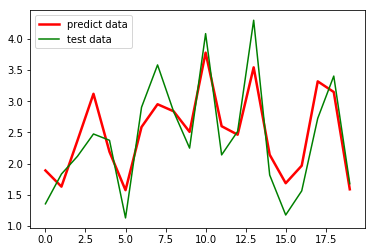效果勉强满意，毕竟才用了80个数训练的模型，要求高的话多用一些数据就好。
除了用 Python 做线性回归，我们还可以使用 SPSS 来做，不用编程，不用各种调参，就是点几下就可以输出结果，更简便！具体操作请参考下面链接里的文章：
数据建模 - SPSS做多元线性回归 - 分析步骤、输出结果详解、与Python的结果对比 -（SPSS）

【此处是重点】打赏一下发数据！


展开全文python 回归分析
• 线性回归输出是一个连续值，因此适用于回归问题。分类问题中模型的最终输出是一个离散值。softmax回归则适用于分类问题。线性回归和softmax回归都是单层神经网络。 线性回归的基本要素 模型定义，其中其中 $和 是... 线性回归输出是一个连续值，因此适用于回归问题。分类问题中模型的最终输出是一个离散值。softmax回归则适用于分类问题。线性回归和softmax回归都是单层神经网络。 线性回归的基本要素 模型定义，其中其中$和 是权重（weight），是偏差（bias）,且均为标量，都是模型的参数。模型输出 是线性回归对真实价格的预测或估计。我们通常允许它们之间有一定误差。
模型训练：需要通过数据来寻找特定的模型参数值，使模型在数据上的误差尽可能小。这个过程叫作模型训练（model training）
训练数据：假设我们采集的样本数为 ，索引为 的样本的特征为  和 ，标签为 。对于索引为  的样本，线性回归模型表达式为 损失函数：在模型训练中，我们需要衡量价格预测值与真实值之间的误差。通常我们会选取一个非负数作为误差，且数值越小表示误差越小。一个常用的选择是平方函数。 它在评估索引为  的样本误差的表达式为 ，通常，我们用训练数据集中所有样本误差的平均来衡量模型预测的质量，即   在模型训练中，我们希望找出一组模型参数，记为 $w_1^, w_2^, b^*$，来使训练样本平均损失最小： 优化算法：当模型和损失函数形式较为简单时，上面的误差最小化问题的解可以直接用公式表达出来。这类解叫作解析解（analytical solution）。本节使用的线性回归和平方误差刚好属于这个范畴。然而，大多数深度学习模型并没有解析解，只能通过优化算法有限次迭代模型参数来尽可能降低损失函数的值。这类解叫作数值解（numerical solution）。在求数值解的优化算法中，小批量随机梯度下降（mini-batch stochastic gradient descent）在深度学习中被广泛使用。它的算法很简单：先选取一组模型参数的初始值，如随机选取；接下来对参数进行多次迭代，使每次迭代都可能降低损失函数的值。在每次迭代中，先随机均匀采样一个由固定数目训练数据样本所组成的小批量（mini-batch），然后求小批量中数据样本的平均损失有关模型参数的导数（梯度），最后用此结果与预先设定的一个正数的乘积作为模型参数在本次迭代的减小量。在训练本节讨论的线性回归模型的过程中，模型的每个参数将作如下迭代：在上式中， 代表每个小批量中的样本个数（批量大小，batch size）， 称作学习率（learning rate）并取正数。需要强调的是，这里的批量大小和学习率的值是人为设定的，并不是通过模型训练学出的，因此叫作超参数（hyperparameter）。我们通常所说的“调参”指的正是调节超参数，例如通过反复试错来找到超参数合适的值。在少数情况下，超参数也可以通过模型训练学出。
模型预测（模型推断、模型测试）
模型训练完成后，我们将模型参数 在优化算法停止时的值分别记作。注意，这里我们得到的并不一定是最小化损失函数的最优解，而是对最优解的一个近似。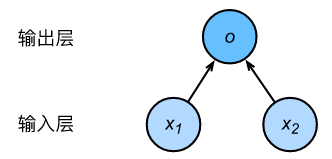输入分别为  和 ，因此输入层的输入个数为2。输入个数也叫特征数或特征向量维度。网络的输出为 ，输出层的输出个数为1。需要注意的是，由于输入层并不涉及计算，按照惯例，图3.1所示的神经网络的层数为1。所以，线性回归是一个单层神经网络。输出层中负责计算 的单元又叫神经元。在线性回归中，的计算依赖于  和 。也就是说，输出层中的神经元和输入层中各个输入完全连接。因此，这里的输出层又叫全连接层（fully-connected layer）或稠密层（dense layer）。

import torch
from IPython import display
from matplotlib import pyplot as plt
import numpy as np
import random

def show(features,labels):
display.set_matplotlib_formats('svg')
plt.rcParams['figure.figsize'] = (3.5,2.5)
plt.scatter(features[:, 1].numpy(), labels.numpy(), 1)
plt.show()

def data_iter(batch_size, features, labels):
# 读取数据
num_examples = len(features)
# print(num_examples)
indices = list(range(num_examples))
random.shuffle(indices)  # 样本的读取顺序是随机的
for i in range(0, num_examples, batch_size):
j = torch.LongTensor(indices[i: min(i + batch_size, num_examples)]) # 最后一次可能不足一个batch
yield  features.index_select(0, j), labels.index_select(0, j)

# 定义模型

def linreg(X, w, b):  # 本函数已保存在d2lzh_pytorch包中方便以后使用
# 定义损失函数
def squared_loss(y_hat, y):  # 本函数已保存在d2lzh_pytorch包中方便以后使用
# 注意这里返回的是向量, 另外, pytorch里的MSELoss并没有除以 2
return (y_hat - y.view(y_hat.size())) ** 2 / 2

# 定义优化算法
def sgd(params, lr, batch_size):  # 本函数已保存在d2lzh_pytorch包中方便以后使用
for param in params:
param.data -= lr * param.grad / batch_size # 注意这里更改param时用的param.data

if __name__ =='__main__':
num_inputs = 2
num_examples = 1000
true_w = [2, -3.4]
true_b = 4.2
# 生成数据集
features = torch.randn(num_examples, num_inputs,
dtype=torch.float32)
# # labels = true_w * features[:, 0] + true_w * features[:, 1] + true_b
labels = torch.mm(features, torch.tensor(true_w).view(2, 1)) + true_b
labels += torch.tensor(np.random.normal(0, 0.01, size=labels.size()),
dtype=torch.float32)
show(features, labels)
# 初始化模型
w = torch.tensor(np.random.normal(0, 0.01, (num_inputs, 1)), dtype=torch.float32)
b = torch.zeros(1, dtype=torch.float32)

lr = 0.03      # 学习率
num_epochs = 3
net = linreg
loss = squared_loss
batch_size = 10

for epoch in range(num_epochs):  # 训练模型一共需要num_epochs个迭代周期
# 在每一个迭代周期中，会使用训练数据集中所有样本一次（假设样本数能够被批量大小整除）。X
# 和y分别是小批量样本的特征和标签
for X, y in data_iter(batch_size, features, labels):
# 由于变量l并不是一个标量，所以我们可以调用.sum()将其求和得到一个标量，再运行l.backward()得到该变量有关模型参数的梯度。
l = loss(net(X, w, b), y).sum()  # l是有关小批量X和y的损失
l.backward()  # 小批量的损失对模型参数求梯度
sgd([w, b], lr, batch_size)  # 使用小批量随机梯度下降迭代模型参数

# 不要忘了梯度清零
train_l = loss(net(features, w, b), labels)
print('epoch %d, loss %f' % (epoch + 1, train_l.mean().item()))
print('true_w:',true_w, '\n', w)
print('true_b:',true_b, '\n', b)
import torch
import numpy as np
import random
import torch.utils.data as Data
from torch import nn
from torch.nn import init
import torch.optim as optim

# 定义模型
class LinearNet(nn.Module):
def __init__(self, n_feature):
super(LinearNet, self).__init__()
self.linear = nn.Linear(n_feature, 1)
# forward 定义前向传播
def forward(self, x):
y = self.linear(x)
return y

if __name__ == '__main__':
# 生产数据集
num_inputs = 2
num_examples = 1000
true_w = [2, -3.4]
true_b = 4.2
features = torch.tensor(np.random.normal(0, 1, (num_examples, num_inputs)), dtype=torch.float)
labels = true_w * features[:, 0] + true_w * features[:, 1] + true_b
labels += torch.tensor(np.random.normal(0, 0.01, size=labels.size()), dtype=torch.float)

# 读取数据集
batch_size = 10
# 将训练数据的特征和标签组合
dataset = Data.TensorDataset(features, labels)
# 随机读取小批量

# net = LinearNet(num_inputs)
# print(net)  # 使用print可以打印出网络的结构
# # 写法一
# net = nn.Sequential(
#     nn.Linear(num_inputs, 1)
#     # 此处还可以传入其他层
# )
#
# # 写法二
# net = nn.Sequential()
#
# # 写法三
from collections import OrderedDict

net = nn.Sequential(OrderedDict([
('linear', nn.Linear(num_inputs, 1))
# ......
]))
#
# print(net)
# print(net)

# 初始化模型参数
init.normal_(net.linear.weight, mean=0, std=0.01)
init.constant_(net.linear.bias, val=0)  # 也可以直接修改bias的data: net.bias.data.fill_(0)

# 损失函数
loss = nn.MSELoss()

# 优化算法
optimizer = optim.SGD(net.parameters(), lr=0.03)

# 训练模型
num_epochs = 3
for epoch in range(1, num_epochs + 1):
for X, y in data_iter:
output = net(X)
l = loss(output, y.view(-1, 1))
l.backward()
optimizer.step()
print('epoch %d, loss: %f' % (epoch, l.item()))
dense = net.linear
print(true_w, dense.weight)
print(true_b, dense.bias)

展开全文深度学习 pytorch
• 一、标准线性回归   在线性回归中我们要求的参数为： 详细的推导可以参见：http://blog.csdn.net/weiyongle1996/article/details/73727505 所以代码实现主要就是实现上式，python代码如下： import ...
• 这一篇文章，我们将首次介绍回归算法即对连续性的数据做出预测。回归一词的来历由来已久。“回归”是由英国著名生物学家兼统计学家高尔顿(Francis Galton,1822～1911.生物学家达尔文的表弟)在研究人类遗传问题时提...lr ridge lasso
• 线性回归 解决回归问题 思想简单，实现容易 许多强大的非线性模型的基础 结果具由很好的可解释性 蕴含机器学习中的很多重要思想 例如 房屋价格（输出标记）和面积（样本特征）之间的关系 简单线性回归 样本特征...
• 线性回归的输出是一个连续值，使用于回归问题（如房价预测、气温预测等）。线性回归可以理解为单层的神经网络。 线性回归的基本要素 本小节以一个简单的房屋价格预测作为例子来解释线性回归的基本要素。此应用的...pytorch 深度学习
• model_lr = LinearRegression() # 建立普通线性回归模型对象 model_etc = ElasticNet() # 建立弹性网络回归模型对象 model_svr = SVR() # 建立支持向量机回归模型对象 model_gbr = GradientBoostingRegressor() # ...LinearSVC 机器学习
• 预测回归二：局部加权回归和惩罚线性回归 预测回归二：局部加权回归和惩罚线性回归 一、局部加权回归 原理 python 代码实现 二、惩罚线性回归 2.1 岭回归 2.2 lasso 缩减法 2.3 ElasticNet 形式化 2.4 求解...机器学习 python
• 线性回归代码。具体要求如下：（1）回归函数为：x^2-0.5 + noisy （2）神经网络的层数、节点数目、激活函数自定义。（记录心得） （3）使用tensorboard把计算图显示出来。 （4）使用matplotlib.pyplot把拟合情况画...
• # 分割自变量 y = raw_data[:, -1] # 分割因变量 # 训练回归模型 n_folds = 6 # 设置交叉检验的次数 model_br = BayesianRidge() # 建立贝叶斯岭回归模型对象 model_lr = LinearRegression() # 建立普通线性回归模型...
• 现在用 Python 写线性回归的博客都快烂大街了，为什么还要用 SPSS 做线性回归呢？这就来说说 SPSS 存在的原因吧。 SPSS 是一个很强大的软件，不用编程，不用调参，点巴两下就出结果了，而且出来的大多是你想要的。...数据建模 Python
• 1 多元线性回归LinearRegression原理建模评估指标2 岭回归建模Ridge专门的选择最好alpha的交叉验证3 Lasso 的核心作用：特征选择4 多项式回归多项式回归提升模型表现 1 多元线性回归LinearRegression 原理 线性回归...python 机器学习
• 线性回归3.1 线性回归3.1.1 线性回归的基本要素3.1.1.1 模型定义3.1.1.2 模型训练(1) 训练数据(2) 损失函数(3) 优化算法3.1.1.3 模型预测3.1.2 线性回归的表示方法3.1.2.1 神经网络图3.1.2.2 矢量计算表达式小结 ...机器学习 神经网络 人工智能
• 线性回归 目录 一、线性回归介绍 二、多元线性回归 1、原理 2、最小二乘法求解多元线性回归的参数 3、sklearn练习 三、回归类模型评估指标 1、是否预测到了正确的数值 2、是否拟合到了足够的信息 四、岭回归和Lasso ...
• 目录1、线性回归简介1.1 线性回归应用场景1.2 什么是线性回归1.2.1 定义与公式1.2.2 线性回归的特征与目标的关系分析2、线性回归api初步使用2.1 线性回归API2.2 举例2.2.1 步骤分析2.2.2 代码过程3、线性回归的损失...
• 线性学习中最基础的回归之一，本文从线性回归的数学假设，公式推导，模型算法以及实际代码运行几方面对这一回归进行全面的剖析~ 一：线性回归的数学假设 1.假设输入的X和Y是线性关系，预测的y与X通过线性方程建立...
• 文章目录一、线性回归原理1.构造模型2.构建损失函数3.优化损失二、设计方案1.准备数据2.构造损失函数3.优化损失三、代码实现四、总结 一、线性回归原理 1.构造模型 y = w1x1+w2x2…+b 2.构建损失函数 均方误差 3....python tensorflow 机器学习
• 分享笔者做线性回归的整体思路：确定因变量是否服从高斯分布；利用显著性检验寻找影响因变量的自变量；对自变量做必要的数据处理；模型建立与迭代评估线性回归模型效果一般用可决系数R2回归模型需要...python 机器学习 人工智能 数据分析 统计学
• 3.1 线性回归 线性回归输出是一个连续值，因此适用于回归问题。回归问题在实际中很常见，如预测房屋价格、气温、销售额等连续值的问题。与回归问题不同，分类问题中模型的最终输出是一个离散值。我们所说的图像分类...神经网络 算法 python 深度学习
• -评估、调优（调参） 普通线性回归 先举个例子，比如有一组数据，美国纽约若干程序员职位的年薪 可以看出，年薪只跟工作经验有关，将数据抽取出来 X代表工作经验，y代表年薪。那x...机器学习 深度学习
• 线性回归原理讲解 定义 回归（regression）是指⼀类为⼀个或多个⾃变量与因变量之间关系建模的⽅法。在⾃然科学和社会科学领域，回归经常⽤来表示输入和输出之间的关系。 线性回归假设 线性回归基于⼏个简单的...深度学习 机器学习
• 回归分析整体逻辑 回归分析（Regression Analysis） ...线性回归分析 - 简单线性回归 - 多重线性回归线性回归分析 - 逻辑回归 - 神经网络 回归分析的步骤 根据预测目标，确定自变量和因变量 绘制散...回归分析...